```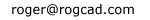----------------

```
`Simple trig`
 home Balloting Simple trig Logistic map The Attractor of Henon Number doubling Barnsley's Fern The Sierpinski Triangle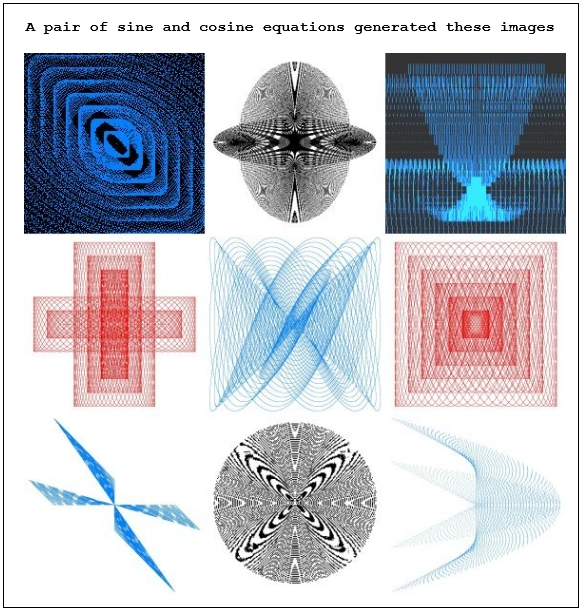``` Simple trig For C = 1 To iterations xangle = xangle + xinc yangle = yangle + yinc RN1 = RN1 + xinc: If RN1 > 1 Then RN1 = 0 RN2 = RN2 + yinc: If RN2 > 1 Then RN2 = 0 X = RN1 * (Cos(xangle) * ra) + 400 Y = RN2 * (Sin(yangle) * ra) + 400 viewport.PSet (X, Y), RGB(rd, gn, bl) Next C ```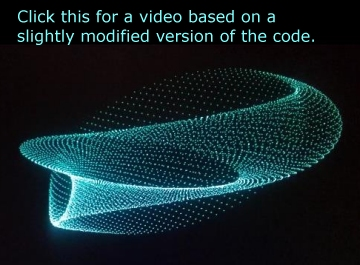``` I played a hunch and came up with an algorithm for a pair of iterated trig equations, with offsetting scalar multipliers for each equation. Elaborate patterns appeared which went way beyond what I could have imagined. This program plots the sine of an incremently increasing angle and the cosine of an incremently increasing angle on the same graph. The user has the option of making those increments the same or different. The user can also specify whether the scalar mulitplier is the same or different for the two equations. The most interesting patterns are generated when the increments are the same or extremely close to the same. Offsetting the scalar multipliers affects the symmetry in interesting ways. 40,000 to 1,000,000 iterations are typically optimum. The complete VisualBASIC program code is displayed further down. (02/04/17 update: I've just begun generating 3D images of these trig functions, which automatically have even more complex shapes. Great stuff. Some of the images have been included at the bottom of this page.) First up, are some spheres with various parameters. Further down, are displayed some very surprising images -- squares, triangles, spirals, and a host of shapes that defy classification. A couple half size images using different parameters: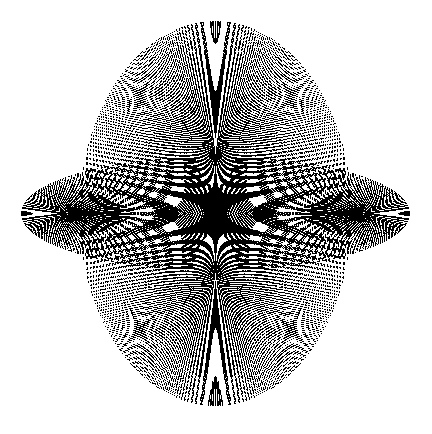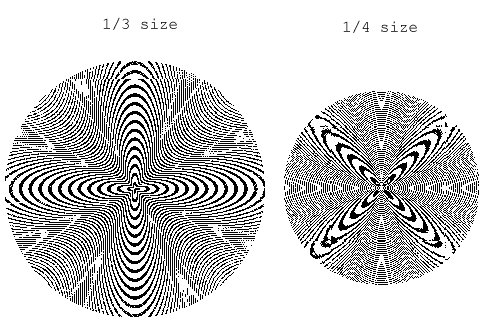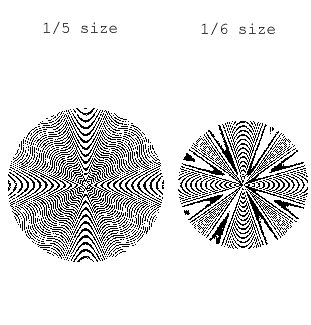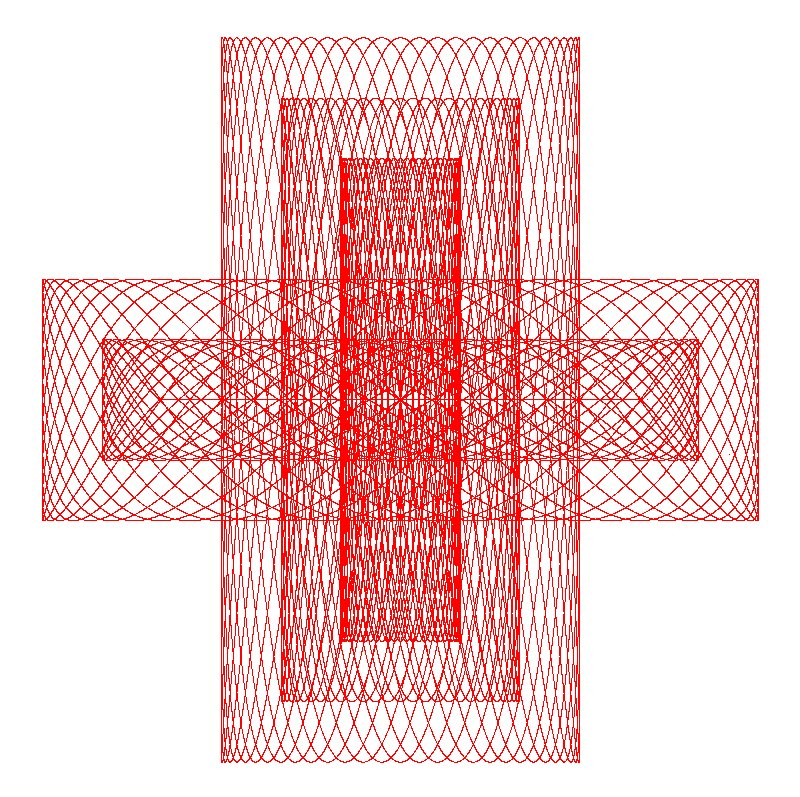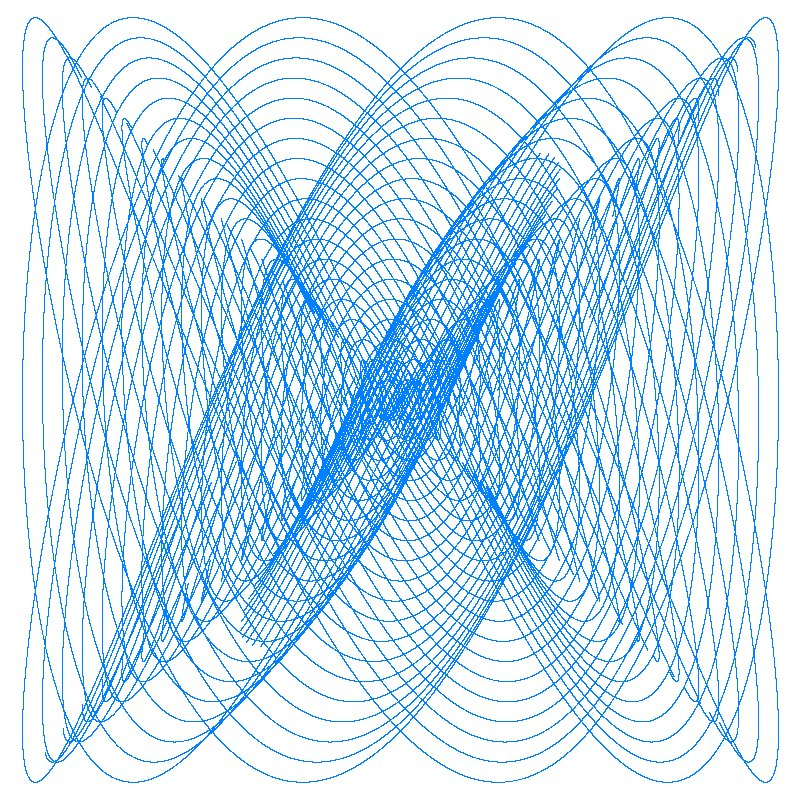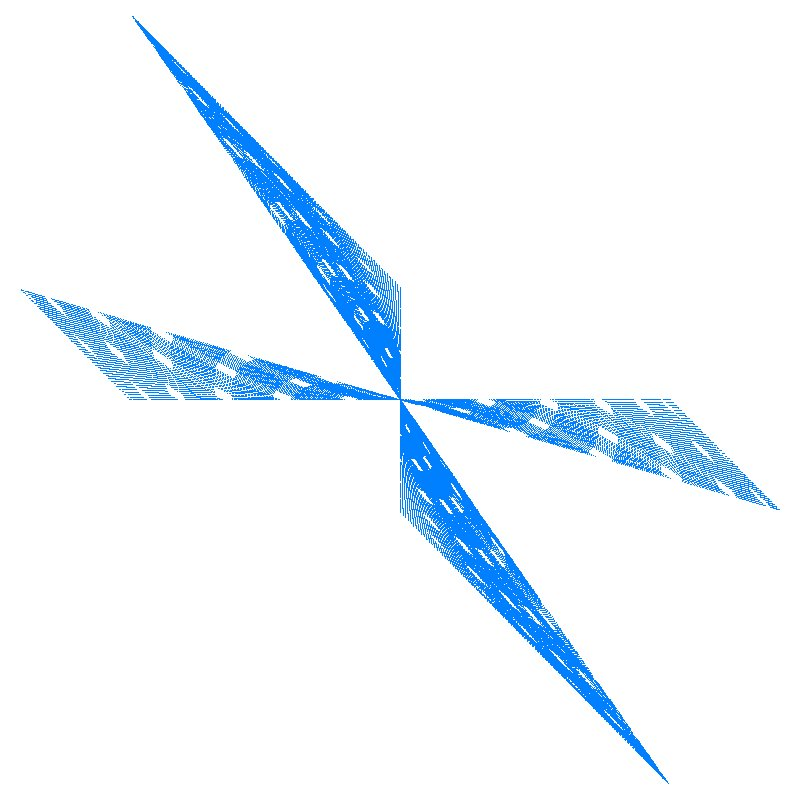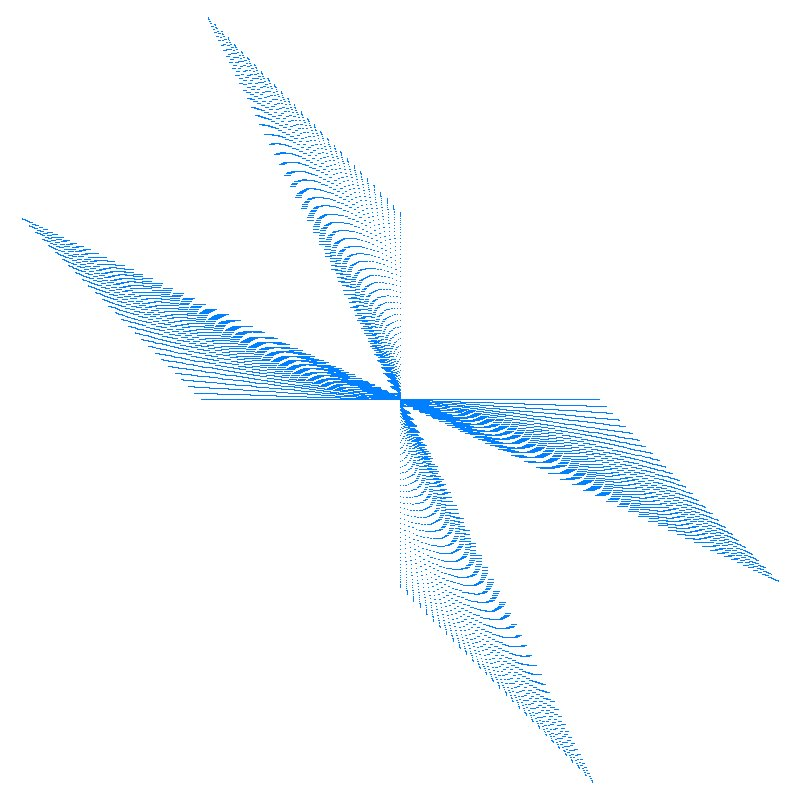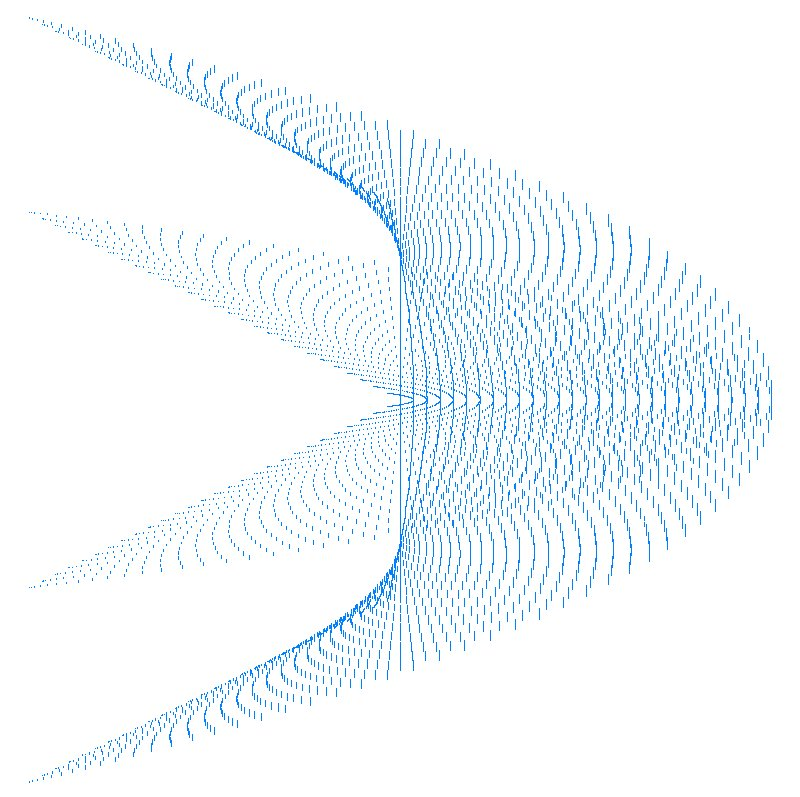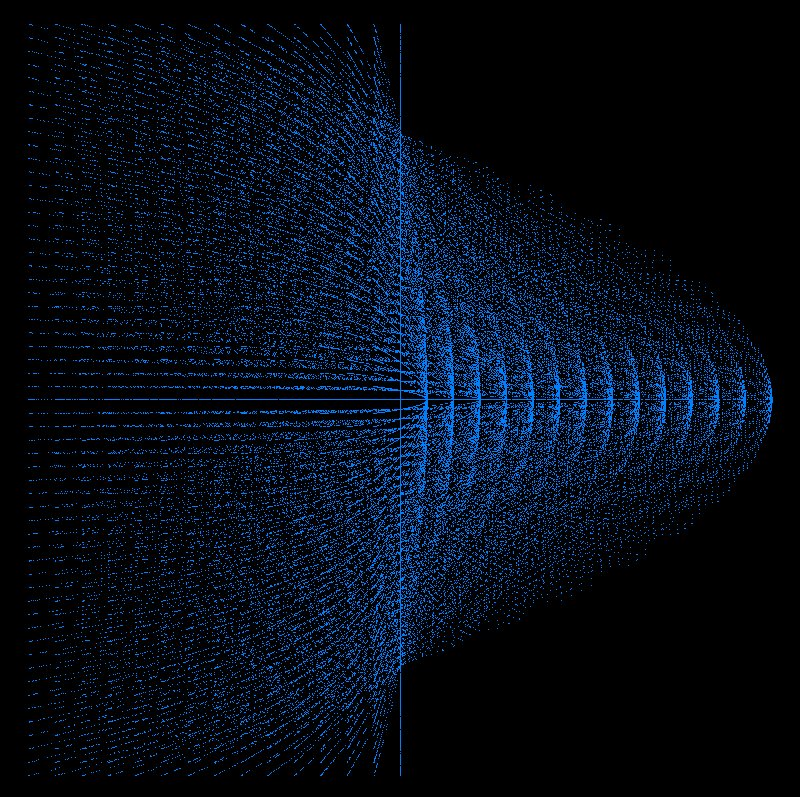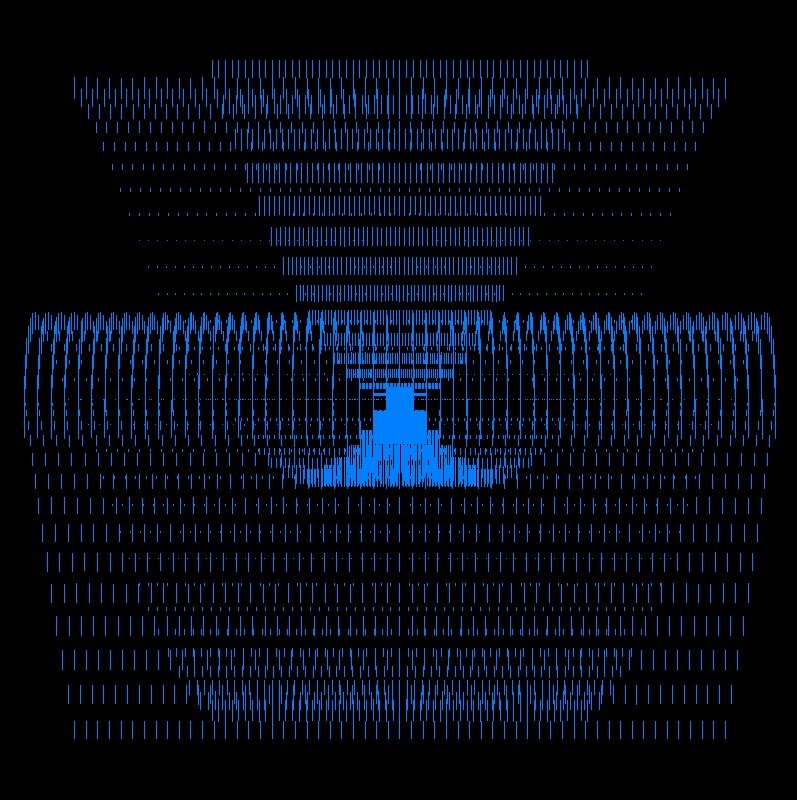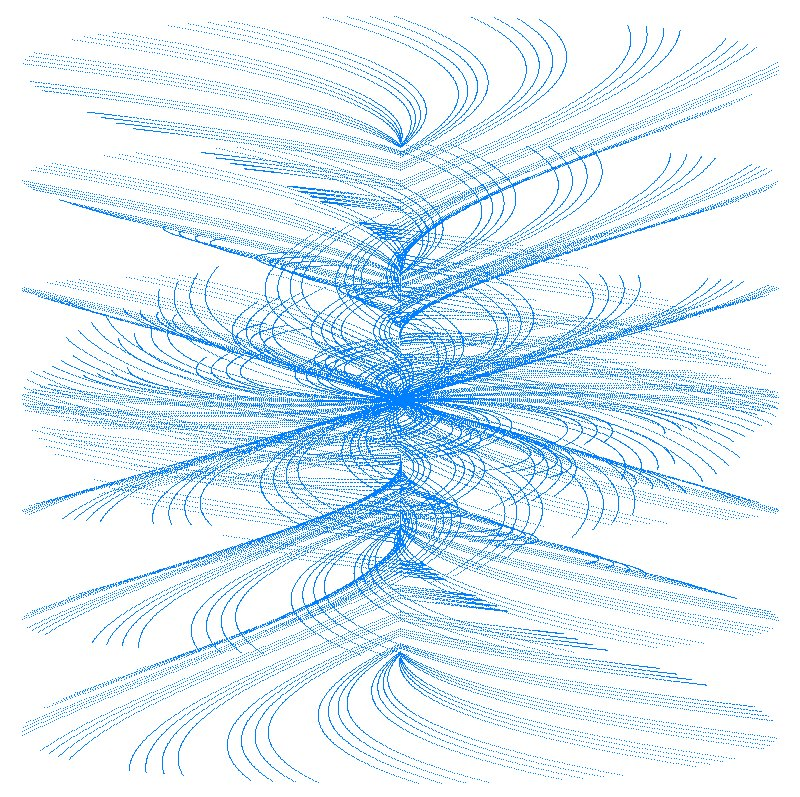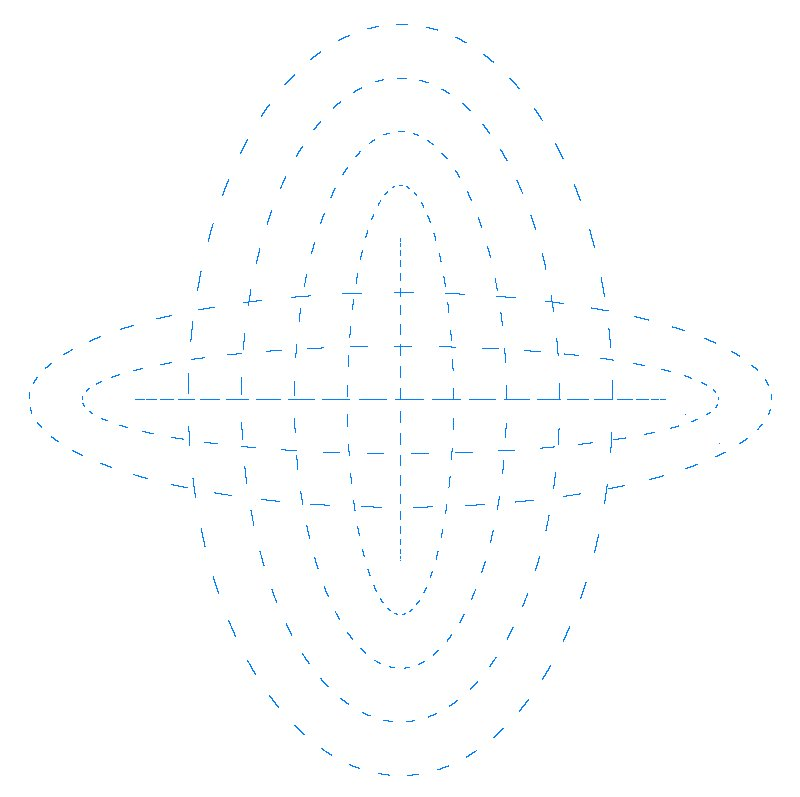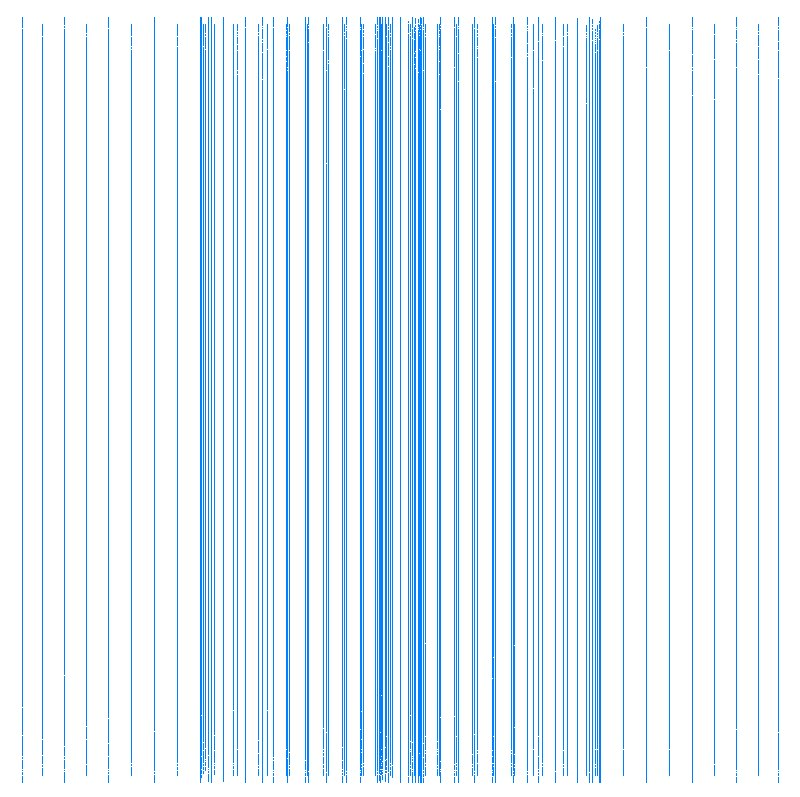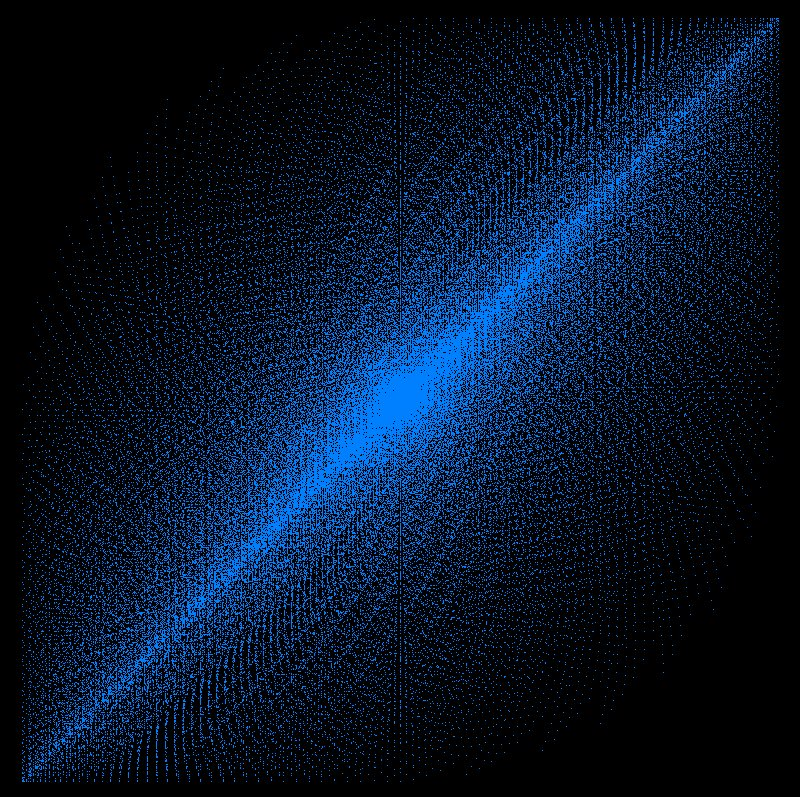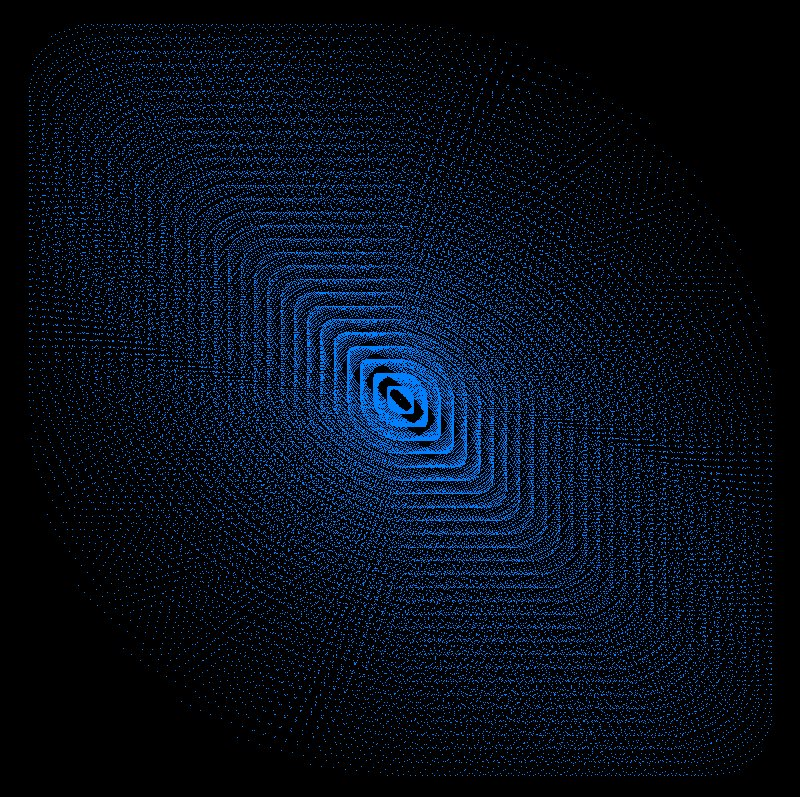``` ``` 3D version images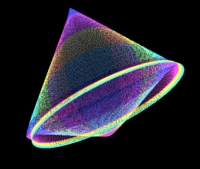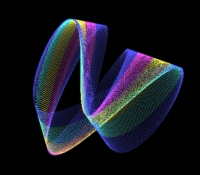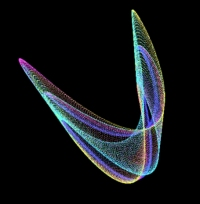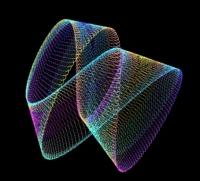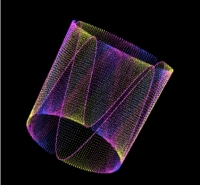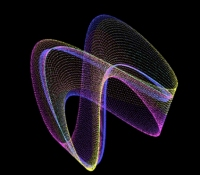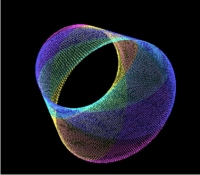```
 ```Here are some 3D images.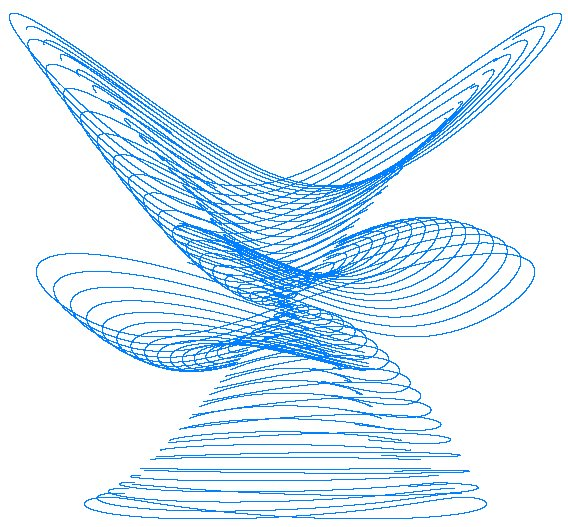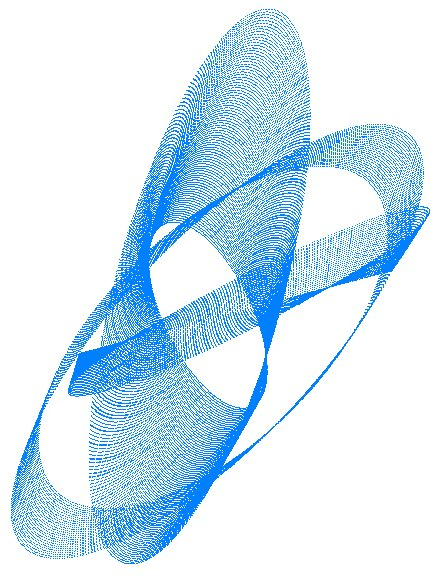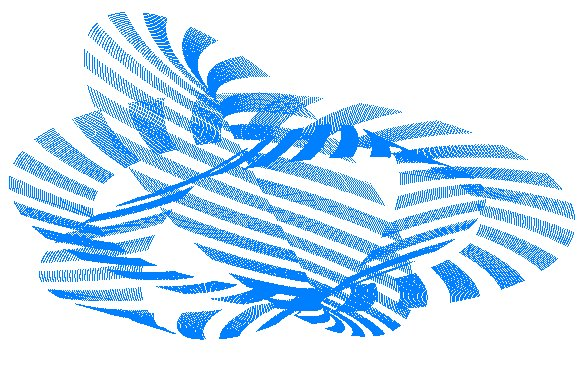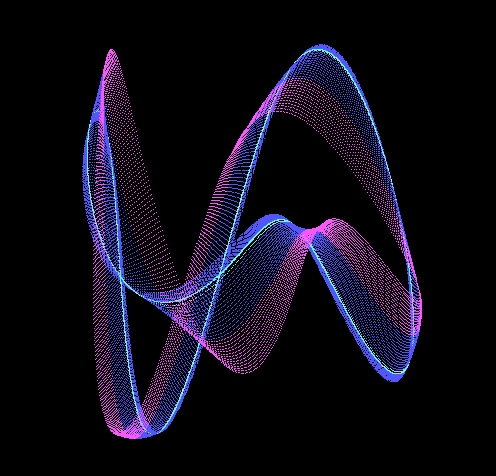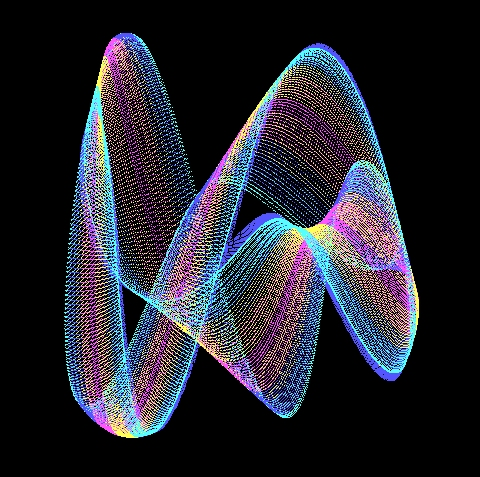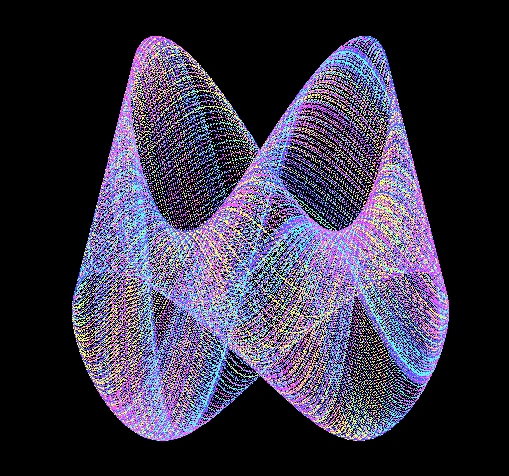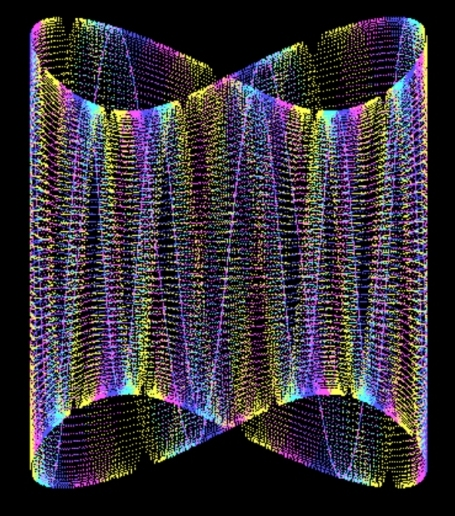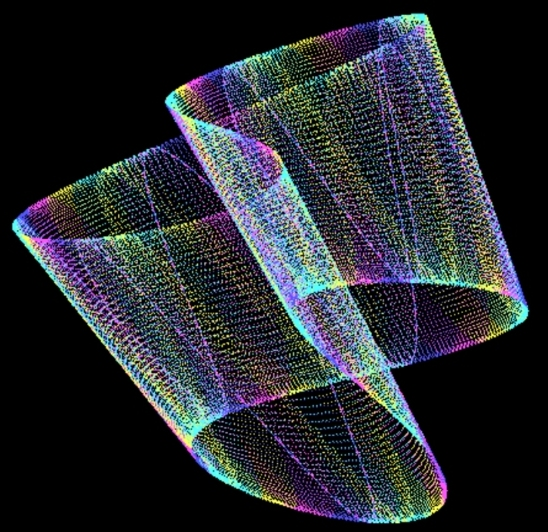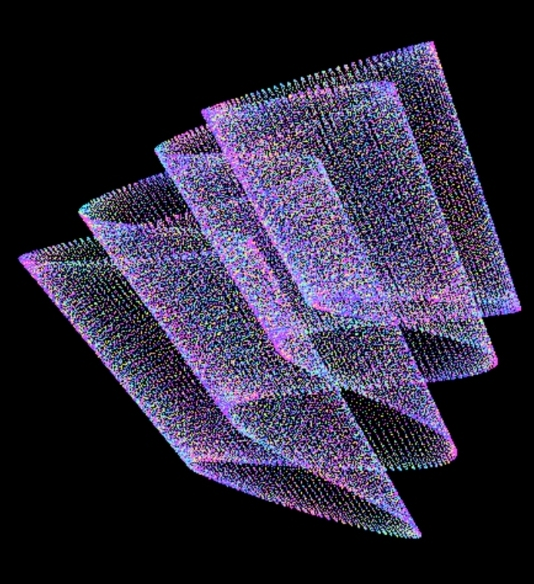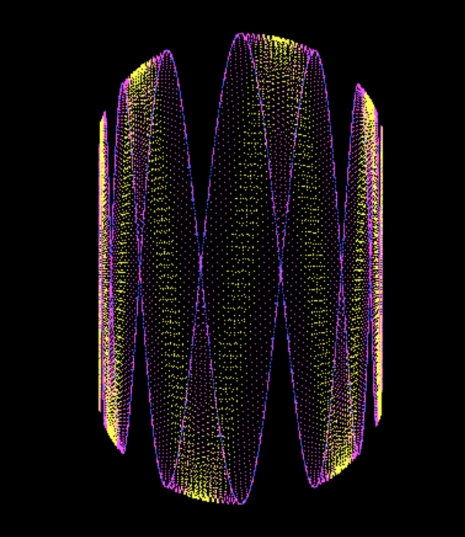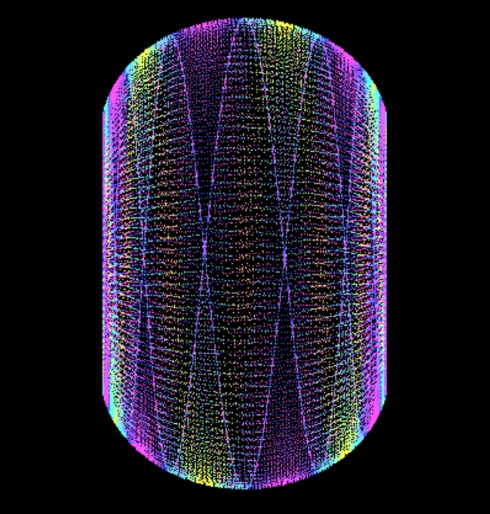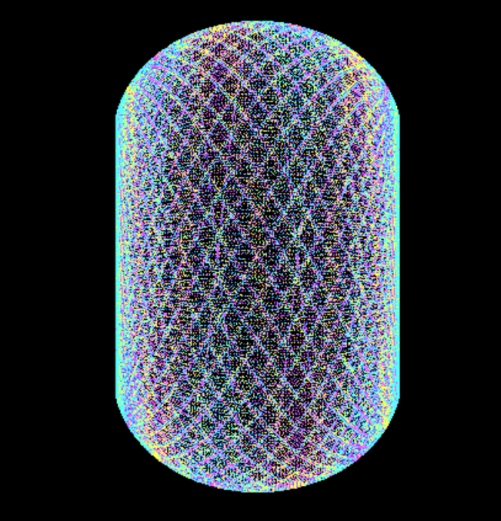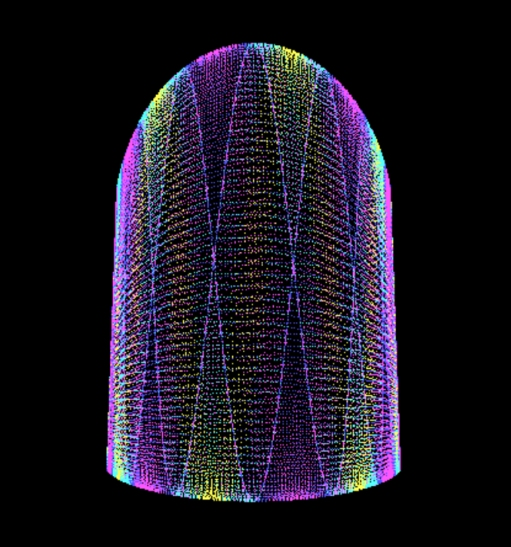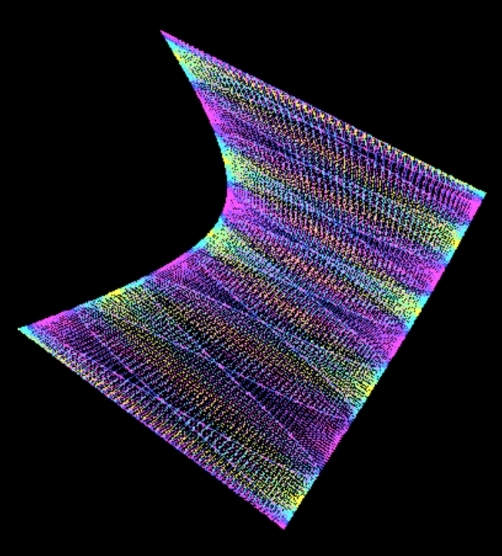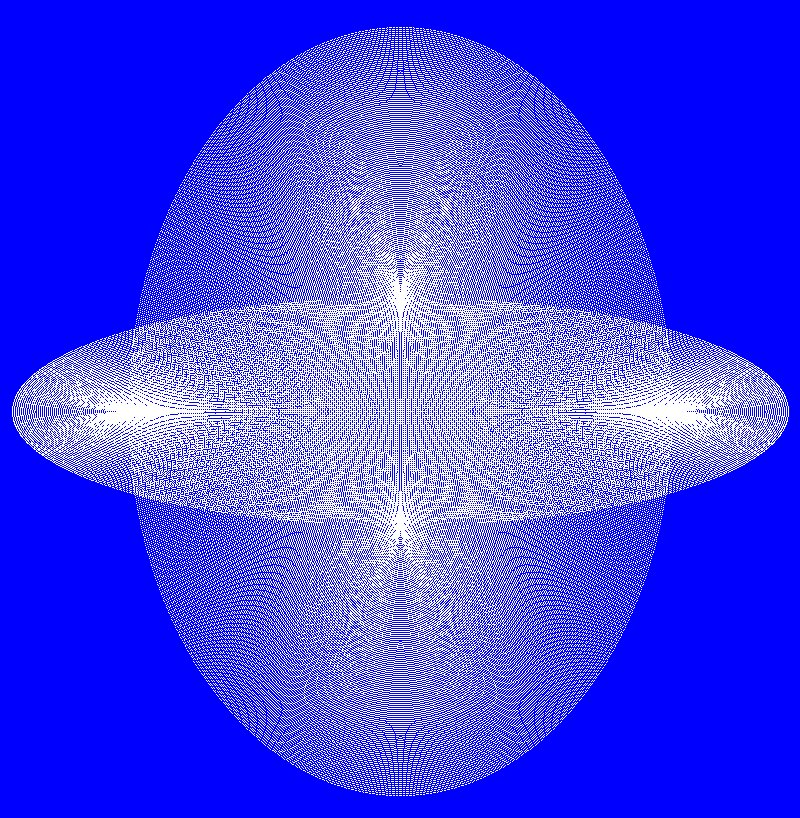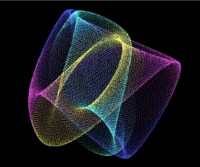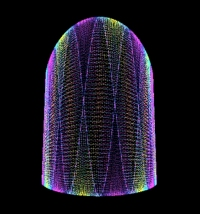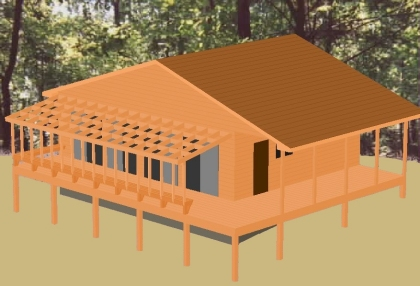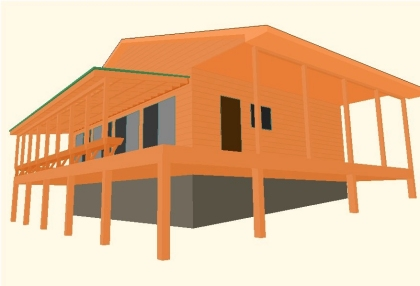``` ``` My VisualBASIC program code: (2D) (3D further down) Private Sub trigrunbtn_Click() iter = Val(iterbox.Text) xinc = Val(xincbox.Text) * (3.14159265358979 / 180) yinc = Val(yincbox.Text) * (3.14159265358979 / 180) RN1 = Val(rn1box.Text) RN2 = Val(rn2box.Text) For C = 1 To iter xangle = xangle + xinc yangle = yangle + yinc RN1 = RN1 + xinc: If RN1 > 1 Then RN1 = 0 RN2 = RN2 + yinc: If RN2 > 1 Then RN2 = 0 X = RN1 * (Cos(xangle) * 380) + 400 'or sine sine Y = RN2 * (Sin(yangle) * 380) + 400 viewport.PSet (X, Y), RGB(redd, greenn, bluee) Next C End Sub ------------ My VisualBASIC program code: (3D) (Includes rotation of image around all three axis) Private Sub trigrunbtn_Click() iter = Val(iterbox.Text) xinc = Val(xincbox.Text) * (3.14159265358979 / 180) yinc = Val(yincbox.Text) * (3.14159265358979 / 180) zinc = Val(zincbox.Text) * (3.14159265358979 / 180) RN1 = Val(rn1box.Text) RN2 = Val(rn2box.Text) RN3 = Val(rn3box.Text) RX = RX * (3.14159 / 180) RY = RY * (3.14159 / 180) RY = RZ * (3.14159 / 180) For C = 1 To iter xangle = xangle + xinc yangle = yangle + yinc zangle = zangle + zinc RN1 = RN1 + R1INC: IF RN1 > 1 THEN RN1 = 0 RN2 = RN2 + R2INC: IF RN2 > 1 THEN RN2 = 0 RN3 = RN3 + R3INC: IF RN3 > 1 THEN RN3 = 0 X = RN1 * SIN(XANGLE) Y = RN2 * COS(YANGLE) Z = RN3 * COS(ZANGLE) Y1 = (Y * COS(RX)) - (Z * SIN(RX)) Z1 = ((Y * SIN(RX)) + (Z * COS(RX))) X1 = X Z2 = ((Z1 * COS(RY)) - (X1 * SIN(RY))) X2 = (Z1 * SIN(RY)) + (X1 * COS(RY)) Y2 = Y1 X3 = (X2 * COS(RZ)) - (Y2 * SIN(RZ)) Y3 = (X2 * SIN(RZ)) + (Y2 * COS(RZ)) Z3 = Z2 GX = (((3.9 * X3) / (4 - Y3)) * RA) + 320 GZ = -(((3.9 * Z3) / (4 - Y3)) * RA) + 260 viewport.PSet (GX, GY), RGB(redd, greenn, bluee) Next C End Sub ==================================================== © RogCAD (rogcad.com) © Roger Luebeck home Balloting Simple trig Logistic map The Attractor of Henon Number doubling Barnsley's Fern The Sierpinski Triangle ```